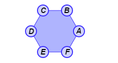# Polygon Angle Sum

### DESCRIPTION

Derive the sum of the angles of a polygon by dividing the polygon into triangles and summing their angles. Vary the number of sides and determine how the sum of the angles changes. Dilate the polygon to see that the sum is unchanged.

Full Lesson Info